Ex 6.1

Chapter 6 Class 12 Application of Derivatives
Serial order wise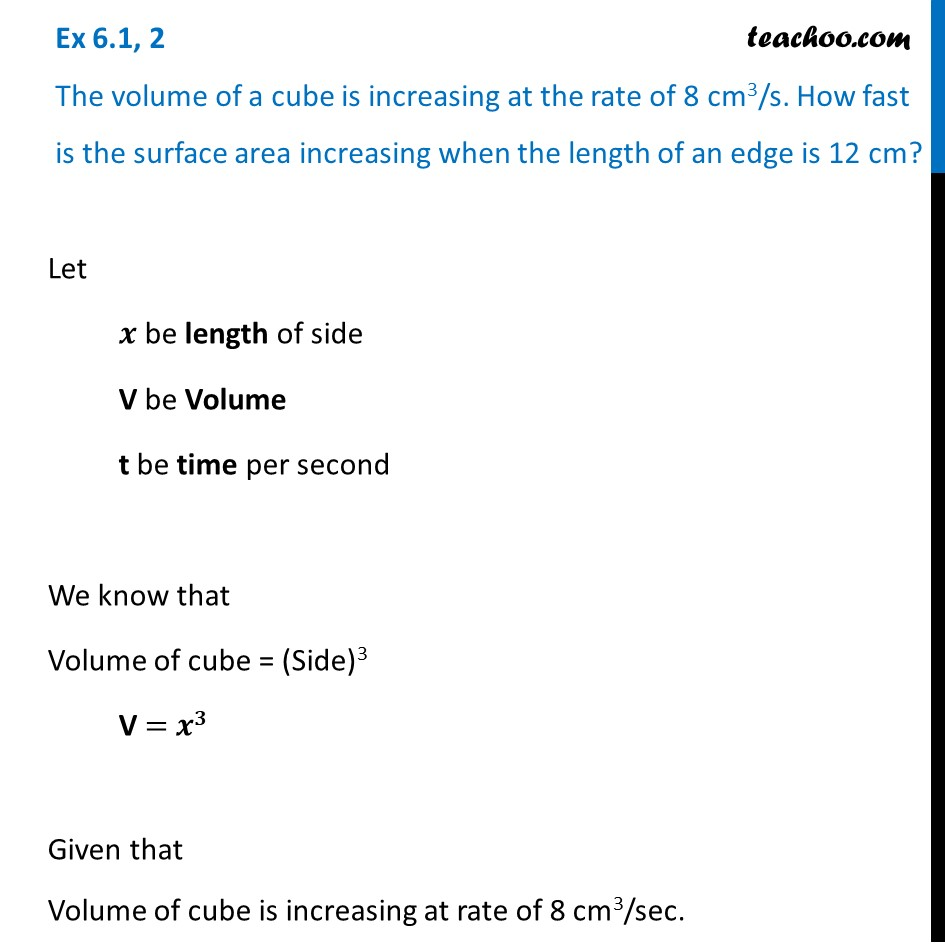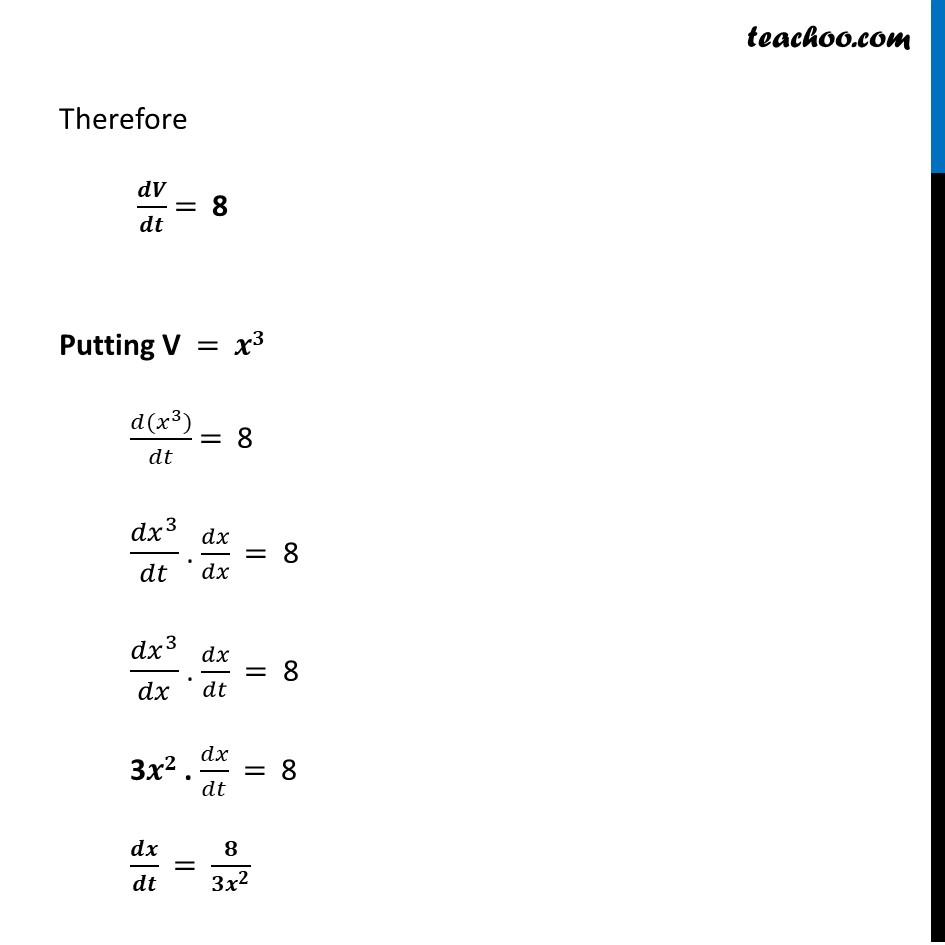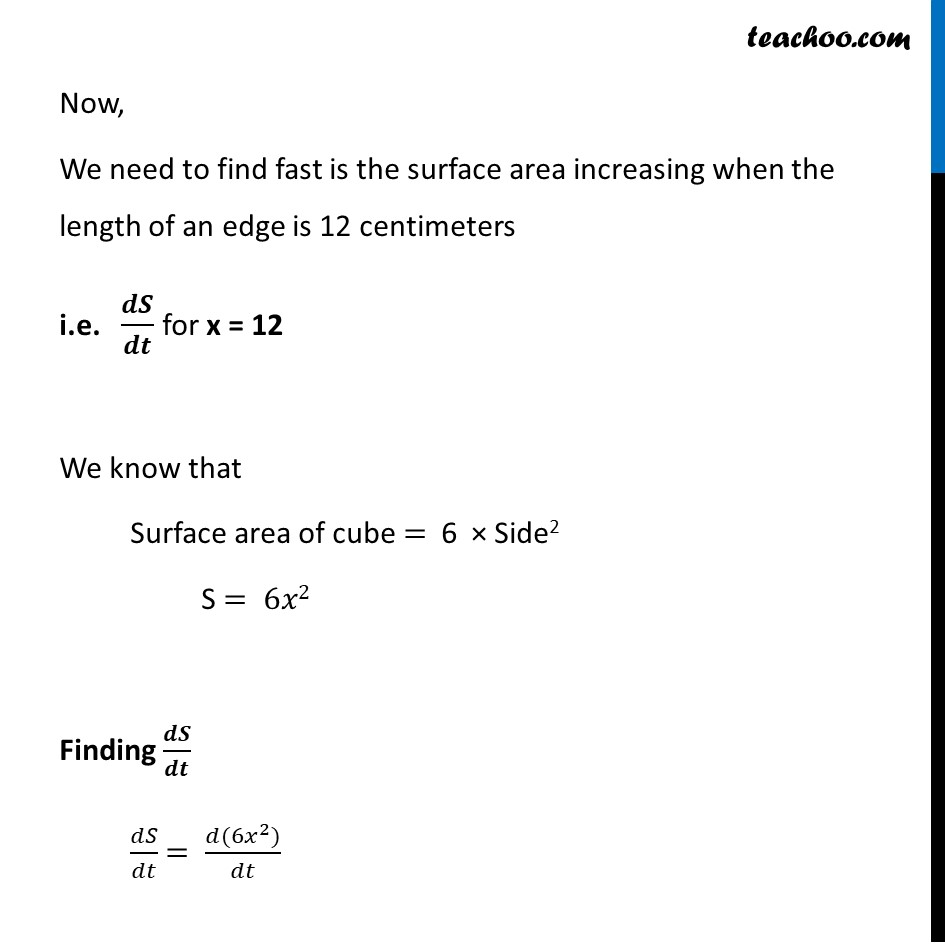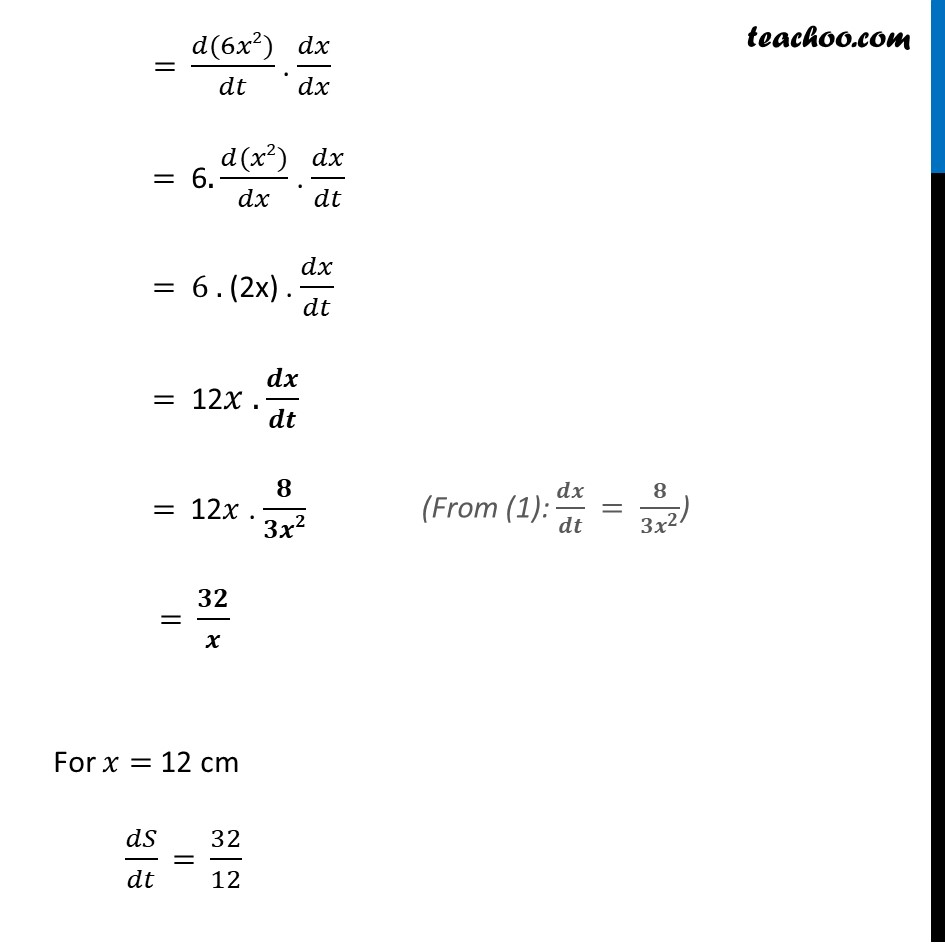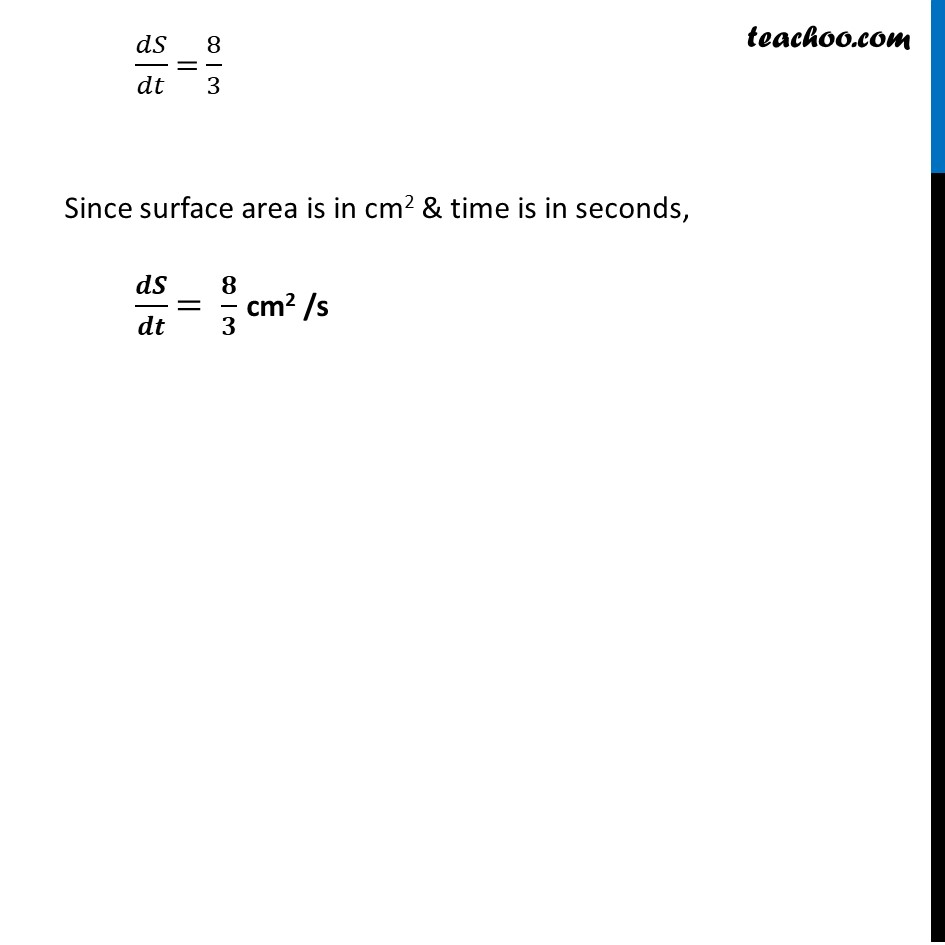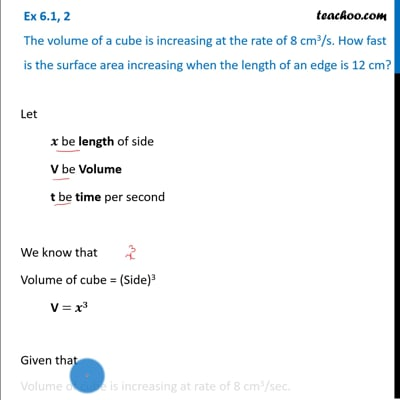This video is only available for Teachoo black users

Solve all your doubts with Teachoo Black (new monthly pack available now!)

### Transcript

Ex 6.1, 2 The volume of a cube is increasing at the rate of 8 cm3/s. How fast is the surface area increasing when the length of an edge is 12 cm?Let 𝒙 be length of side V be Volume t be time per second We know that Volume of cube = (Side)3 V = 𝒙𝟑 Given that Volume of cube is increasing at rate of 8 cm3/sec. Therefore 𝒅𝑽/𝒅𝒕 = 8 Putting V = 𝒙𝟑 (〖𝑑(𝑥〗^3))/𝑑𝑡 = 8 〖𝑑𝑥〗^3/𝑑𝑡 . 𝑑𝑥/𝑑𝑥 = 8 〖𝑑𝑥〗^3/𝑑𝑥 . 𝑑𝑥/𝑑𝑡 = 8 3𝒙𝟐 . 𝑑𝑥/𝑑𝑡 = 8 𝒅𝒙/𝒅𝒕 = 𝟖/〖𝟑𝒙〗^𝟐 Now, We need to find fast is the surface area increasing when the length of an edge is 12 centimeters i.e. 𝒅𝑺/𝒅𝒕 for x = 12 We know that Surface area of cube = 6 × Side2 S = 6𝑥2 Finding 𝒅𝑺/𝒅𝒕 𝑑𝑆/𝑑𝑡 = (𝑑(6𝑥^2))/𝑑𝑡 = (𝑑(6𝑥2))/𝑑𝑡 . 𝑑𝑥/𝑑𝑥 = 6. (𝑑(𝑥2))/𝑑𝑥 . 𝑑𝑥/𝑑𝑡 = 6 . (2x) . 𝑑𝑥/𝑑𝑡 = 12𝑥 . 𝒅𝒙/𝒅𝒕 = 12𝑥 . 𝟖/𝟑𝒙𝟐 = 𝟑𝟐/𝒙 For 𝑥= 12 cm 𝑑𝑆/𝑑𝑡 = 32/12 (From (1): 𝒅𝒙/𝒅𝒕 = 𝟖/(𝟑𝒙^𝟐 )) 𝑑𝑆/𝑑𝑡 = 8/3 Since surface area is in cm2 & time is in seconds, 𝒅𝑺/𝒅𝒕 = 𝟖/𝟑 cm2 /s